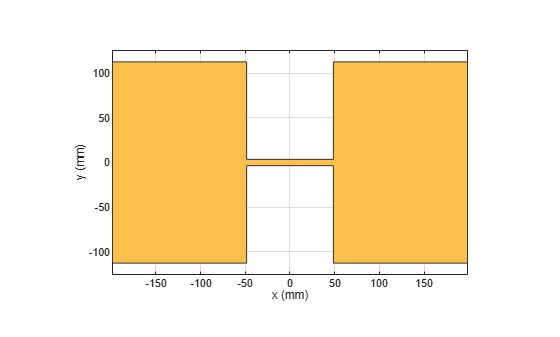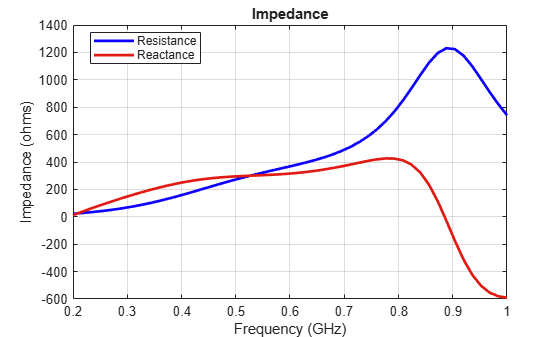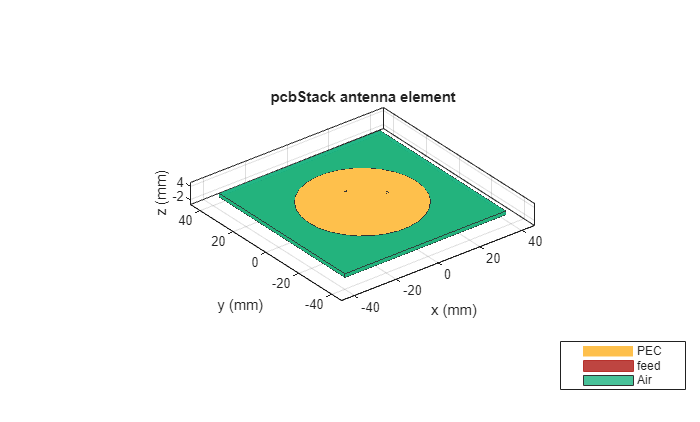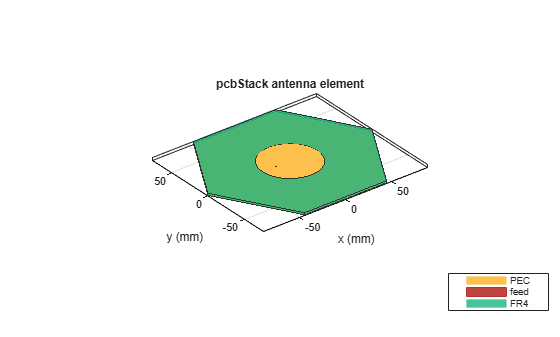# pcbStack

Single-feed or multifeed PCB antenna

## Description

The `pcbStack` object is a single-feed or multi-feed printed circuit board (PCB) antenna. Using the PCB stack, you can create antennas using single-layer or multilayer metal or metal-dielectric substrates. You can also use the PCB stack to create antennas with an arbitrary number of feeds and vias. You can also use Antenna Toolbox™ catalog antennas to create a PCB antenna.

### Note

You require a substrate layer to generate a Gerber file. Use the `Substrate` property to create this layer for the PCB antenna.

## Creation

### Syntax

``pcbant = pcbStack``
``pcbant = pcbStack(Name,Value)``
``pcbant = pcbStack(ant)``

### Description

``` `pcbant = pcbStack` creates an air-filled single-feed PCB with two metal layers.```

example

``` `pcbant = pcbStack(Name,Value)` creates a PCB antenna, with additional properties specified by one or more name-value pair arguments. `Name` is the property name and `Value` is the corresponding value. You can specify several name-value pair arguments in any order as `Name1`, `Value1`, `...`, `NameN`, `ValueN`. Properties not specified retain their default values.```

example

``` `pcbant = pcbStack(ant)` converts any 2-D or 2.5D antenna from the antenna catalog into a PCB antenna for further modeling and analysis.```

## Properties

expand all

Name of PCB antenna, specified a character vector.

Example: `'Name','PCBPatch'`

Data Types: `char`

Revision details of PCB antenna design, specified as a character vector.

Example: `'Revision','2.0'`

Data Types: `char` | `string`

Shape of PC board, specified as an object. The shape can be a rectangle or a polygon.

Example: `'BoardShape',antenna.Polygon`

Thickness of PC board, specified as a positive scalar.

Example: `'BoardThickness',0.02000`

Data Types: `double`

Metal and dielectric layers, specified a cell array of metal layer shapes and dielectric. You can specify one metal shape or one dielectric per layer starting with the top layer and proceeding down.

Data Types: `double`

Feed locations for PCB antenna in Cartesian coordinates, specified as N -by-3 or N-by-4 array. You can place feed inside the board or at the edge of the board. The arrays translate to the following:

• N -by-3 – [x, y, Layer]

• N-by-4 – [x, y, SigLayer, GndLayer]

Example: `'FeedLocations',[-0.0187 0 1 2]`

Data Types: `double`

Center pin diameter of feed connector, specified as a positive scalar in meters.

Example: `'FeedDiameter',2.000e-04`

Data Types: `double`

Electrical short locations for antenna in Cartesian coordinates, specified as a real vector of size M-by-4 array. The arrays translate to the following:

• M-by-4 – [x, y, SigLayer, GndLayer]

Example: `'ViaLocations',[0 -0.025 1 2]`

Data Types: `double`

Electrical shorting pin diameter between metal layers, specified a positive scalar in meters.

Example: `'ViaDiameter',1.0e-3`

Data Types: `double`

Magnitude voltage applied at the feeds, specified as a positive scalar in volts.

Example: `'FeedVoltage',2`

Data Types: `double`

Model for approximating feed and via, specified as one of the following:

• `'strip'` – A rectangular strip approximation to the feed or via cylinder. This approximation is the simplest and results in a small mesh.

• `'square'` – A 4-sided polyhedron approximation to the feed or via cylinder.

• `'hexagon'` – A 6-sided polyhedron approximation to the feed or via cylinder.

• `'octagon'` – A 8-sided polyhedron approximation to the feed or via cylinder.

Example: `'FeedViaModel','octagon'`

Data Types: `double`

Excitation phase at each feed, specified as a real vector in degrees.

Example: `'FeedPhase',2`

Data Types: `double`

Lumped elements added to the antenna feed, specified as a lumped element object handle. For more information, see `lumpedElement`.

Example: `'Load',lumpedelement`. `lumpedelement` is the object handle for the load created using `lumpedElement`.

Example: ```pcbant.Load = lumpedElement('Impedance',75)```

Tilt angle of the antenna, specified as a scalar or vector with each element unit in degrees. For more information, see Rotate Antennas and Arrays.

Example: `'Tilt',90`

Example: `ant.Tilt = 90`

Example: `'Tilt',[90 90]`,`'TiltAxis',[0 1 0;0 1 1]` tilts the antenna at 90 degrees about the two axes, defined by vectors.

### Note

The `wireStack` antenna object only accepts the dot method to change its properties.

Data Types: `double`

Tilt axis of the antenna, specified as:

• Three-element vectors of Cartesian coordinates in meters. In this case, each vector starts at the origin and lies along the specified points on the X-, Y-, and Z-axes.

• Two points in space, each specified as three-element vectors of Cartesian coordinates. In this case, the antenna rotates around the line joining the two points in space.

• A string input describing simple rotations around one of the principal axes, 'X', 'Y', or 'Z'.

Example: `'TiltAxis',[0 1 0]`

Example: `'TiltAxis',[0 0 0;0 1 0]`

Example: `ant.TiltAxis = 'Z'`

### Note

The `wireStack` antenna object only accepts the dot method to change its properties.

## Object Functions

 `show` Display antenna or array structure; display shape as filled patch `info` Display information about antenna or array `axialRatio` Axial ratio of antenna `beamwidth` Beamwidth of antenna `charge` Charge distribution on metal or dielectric antenna or array surface `current` Current distribution on metal or dielectric antenna or array surface `design` Design prototype antenna or arrays for resonance at specified frequency `EHfields` Electric and magnetic fields of antennas; Embedded electric and magnetic fields of antenna element in arrays `impedance` Input impedance of antenna; scan impedance of array `mesh` Mesh properties of metal or dielectric antenna or array structure `meshconfig` Change mesh mode of antenna structure `pattern` Radiation pattern and phase of antenna or array; Embedded pattern of antenna element in array `patternAzimuth` Azimuth pattern of antenna or array `patternElevation` Elevation pattern of antenna or array `returnLoss` Return loss of antenna; scan return loss of array `sparameters` S-parameter object `show` Display antenna or array structure; display shape as filled patch `vswr` Voltage standing wave ratio of antenna `plot` Plot boundary of shape `layout` Display array or PCB stack layout

## Examples

collapse all

Setup parameters.

```vp = physconst('lightspeed'); f = 850e6; lambda = vp./f;```

```L = 0.15; W = 1.5*L; stripL = L; gapx = .015; gapy = .01; r1 = antenna.Rectangle('Center',[0,0],'Length',L,'Width',W,'Center',[lambda*0.35,0]); r2 = antenna.Rectangle('Center',[0,0],'Length',L,'Width',W,'Center',[-lambda*0.35,0]); r3 = antenna.Rectangle('Length',0.5*lambda,'Width',0.02*lambda,'NumPoints',2); s = r1 + r2 + r3; figure show(s)```Assign the radiator shape to pcbStack and make the changes to the board shape and feed diameter properties.

```boardShape = antenna.Rectangle('Length',0.6,'Width',0.3); p = pcbStack; p.BoardShape = boardShape; p.Layers = {s}; p.FeedDiameter = .02*lambda/2; p.FeedLocations = [0 0 1]; figure show(p)```Analyze the impedance of the antenna. Effect of the end-loading should result in the series resonance to be pushed lower in the band.

```figure impedance(p,linspace(200e6,1e9,51))```Create a pcb stack antenna with 2 mm dielectric thickness at the radiator and air below it. Display the structure.

```p = pcbStack; d1 = dielectric('FR4'); d1.Thickness = 2e-3; d2 = dielectric('Air'); d2.Thickness = 8e-3; p.Layers = {p.Layers{1},d1,d2,p.Layers{2}}; p.FeedLocations(3:4) = [1 4]; show(p)```Create a PCB stack antenna from reflector backed bowtie.

```b = design(bowtieRounded,1e9); b.Tilt = 90```
```b = bowtieRounded with properties: Length: 0.0959 FlareAngle: 90 Tilt: 90 TiltAxis: [1 0 0] Load: [1x1 lumpedElement] ```
```b.TiltAxis = [0 1 0]; r = reflector('Exciter',b); p = pcbStack(r);```

Plot the directivity pattern of the antenna at 1 GHz.

`pattern(p,1e9);`Create a coplanar inverted F antenna.

```fco = invertedFcoplanar('Height',14e-3,'GroundPlaneLength', 100e-3, ... 'GroundPlaneWidth', 100e-3);```

Use this antenna to create a `pcbStack` object.

`p = pcbStack(fco);`

Create a circular microstrip patch.

```p = patchMicrostripCircular; d = dielectric; d.EpsilonR = 4.4; p.Radius = .0256; p.Height = 1.6e-3; p.Substrate = d; p.GroundPlaneLength = 3*.0256; p.GroundPlaneWidth = 3*.0256; p.FeedOffset = [.0116 0]; ```

Create a PCB circular microstrip patch using `pcbStack`.

```pb = pcbStack(p); pb.FeedDiameter = 1.27e-3; pb.ViaLocations = [0 pb.FeedLocations(1)/1.1 1 3]; pb.ViaDiameter = pb.FeedDiameter; figure show(pb) ``````C = SMA_Jack_Cinch; O = PCBServices.MayhewWriter; O.DefaultViaDiam = pb.ViaDiameter; O.Filename = 'Microstrip circular patch-9a'; Am = PCBWriter(pb,O,C); gerberWrite(Am) ```

Images using Mayhew Labs 3-D Viewer.Create a circular microstrip patch antenna.

```ant = design(patchMicrostripCircular,3e9); ant.Substrate = dielectric( 'FR4' ); show(ant)``````c = antenna.Circle; show(c)``````c.NumPoints = 6; c.Radius = 3*ant.Radius; figure show(c)```Create the PCB stack using the vertices derived from the circle shape.

```v = getShapeVertices(c); cp = antenna.Polygon( 'Vertices' ,v); pb = pcbStack(ant); pb.Layers{3} = cp; pb.BoardShape = cp; show(pb) axis equal```Balanis, C. A. Antenna Theory. Analysis and Design. 3rd Ed. Hoboken, NJ: John Wiley & Sons, 2005.

 Stutzman, W. L. and Gary A. Thiele. Antenna Theory and Design. 3rd Ed. River Street, NJ: John Wiley & Sons, 2013.